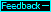Newton's second law of motion      When Isaac Newton was formulating his laws of motion there was no reason for him to suspect that a body's mass changes as its velocity changes. Therefore, his second law (f=m·a), which says that a body's acceleration (a) is proportional to the force (f) causing the acceleration and inversely proportional to the body's mass (m), needs to be modified as follows, according to the quantum medium view.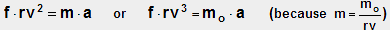This modification reflects the fact that, as a force increases a body's absolute velocity and mass, an increasing part of the force goes into increasing the mass and a decreasing part goes into increasing the velocity. We show this by conducting an imaginary experiment in which a force of 10 absolute newtons (Na) accelerates a mass of 1 kilogram (kg) from va=0 to va=.6 ca. Because rv is changing during the acceleration process, we will break the process into 1 sa time increments. For each time increment we will use the starting velocity to calculate rv and then calculate the body's absolute mass, the acceleration (via the above equation), and the velocity at the end of the time increment. We will repeat this until we get to a velocity of .6 ca or 1.8E8 ma/sa. During the process we will keep track of the distance moved and the work done by the force. Work (W) equals force (f) times distance moved (D).The table below reflects what occurs during the process. In row 1 it shows that after 1 sa the body has a velocity of 10 ma/sa and has moved a distance D of 5 ma and that 50 absolute joules (Ja) (or 50 Na·ma) of work have been done by the 10 Na force. After 300015 sa the body has a velocity of 3E6 ma/sa (or .01 ca) and has moved 1500 LS (roughly the diameter of Mars' orbit). When va is 3E7 ma/sa (.1 ca) the change in rv is significant and the body's mass is 1.005 kga. When the body finally reaches va=.6 ca after 22.5 million sa (about 9 months), it has moved 7.5E6 LS and has a mass of 1.25 kga. The work done on the body is 2.25E16 Ja or .25 kga (because 1 kga=9E16 Ja).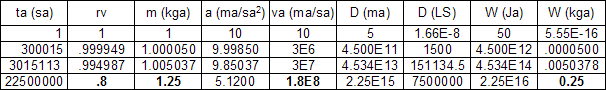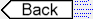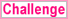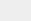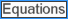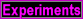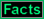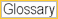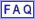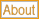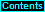To page: 1 2 3 3a 4 5 6 7 8 9 10 11 12 13 14 15 16 17 18 19 20 21 22 23 24 25 26 27 28 29 29a 30 31 32